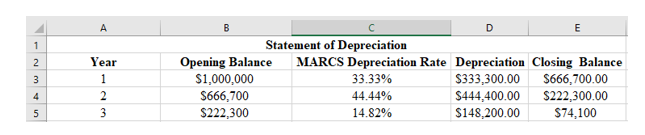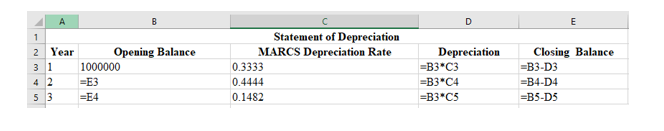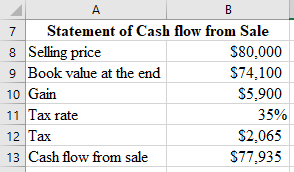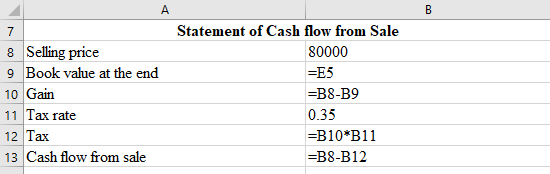# AAA Shop is considering a project to improve their production efficiency. They are trying to decide whether it is a good idea or not to buy an automated injection molding machine which will result in reducing pre-tax costs by \$500,000 for each of the next three years. The molding machine will cost \$1,000,000 and the IRS says it must be depreciated as 3 year MACRS equipment. AAA Shop believes they can sell the machine for \$80,000 at the end of three years. The molding machine will require an initial investment to increase inventoru by \$34,000, and then an additional inventory increase of \$3,000 for each succeeding year of the project. AAA's tax rate is 35% and uses a discount rate of 14%. AAA already spent \$5,000 investigating the feasibility of using the machine. Please show your detailed work so that the NPV of the project is \$52,215.89. What is the \$5000 that AAA already spent investigating the feasibility of using the machine? What is the change in networking capital at the end of the project? What are the depreciations every year? What is the book value of the machine at the end of the project! What is the aftertax salvage value of the equipment at the end of the project? What are the operating cash flows every year? What are the cash flows from assets every year? What is the IRR of the project? Should AAA shop accept the project? Why? MARCS depreciation 3 year 33.33% 44.44% 14.82%

Question
3 views

AAA Shop is considering a project to improve their production efficiency. They are trying to decide whether it is a good idea or not to buy an automated injection molding machine which will result in reducing pre-tax costs by \$500,000 for each of the next three years. The molding machine will cost \$1,000,000 and the IRS says it must be depreciated as 3 year MACRS equipment. AAA Shop believes they can sell the machine for \$80,000 at the end of three years. The molding machine will require an initial investment to increase inventoru by \$34,000, and then an additional inventory increase of \$3,000 for each succeeding year of the project. AAA's tax rate is 35% and uses a discount rate of 14%. AAA already spent \$5,000 investigating the feasibility of using the machine. Please show your detailed work so that the NPV of the project is \$52,215.89. What is the \$5000 that AAA already spent investigating the feasibility of using the machine? What is the change in networking capital at the end of the project? What are the depreciations every year? What is the book value of the machine at the end of the project! What is the aftertax salvage value of the equipment at the end of the project? What are the operating cash flows every year? What are the cash flows from assets every year? What is the IRR of the project? Should AAA shop accept the project? Why? MARCS depreciation 3 year 33.33% 44.44% 14.82%

check_circle

Step 1

The calculation of the depreciation as follows:The formulae used for the calculation of the depreciation as follows:Step 2

The calculation of the cash flow from sale as follows:The formulae used for the calculation of the cash flow from sale as follows:Step 3

The calculation of operating cash flow  as follows:The formulae used for the calculation of the operating cash flow as follows:...

### Want to see the full answer?

See Solution

#### Want to see this answer and more?

Solutions are written by subject experts who are available 24/7. Questions are typically answered within 1 hour.*

See Solution
*Response times may vary by subject and question.
Tagged in